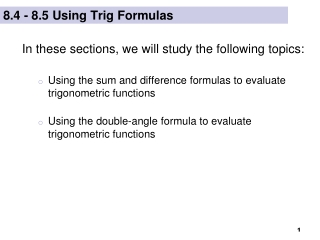DownloadDownload Presentation8.4 - 8.5 Using Trig Formulas

# 8.4 - 8.5 Using Trig Formulas

Télécharger la présentation## 8.4 - 8.5 Using Trig Formulas

- - - - - - - - - - - - - - - - - - - - - - - - - - - E N D - - - - - - - - - - - - - - - - - - - - - - - - - - -
##### Presentation Transcript

1. 8.4 - 8.5 Using Trig Formulas In these sections, we will study the following topics: Using the sum and difference formulas to evaluate trigonometric functions Using the double-angle formula to evaluate trigonometric functions

2. The trig formulas that we will study in sections 8.4 and 8.5 are frequently used in calculus to rewrite trigonometric expressions in a form that helps you to simplify expressions and solve equations. In this course, we will not study the derivations of these formulas, but rather we will use them to evaluate specific trig functions and simplify trig expressions.

3. PLEASE NOTE THAT  The same is true for the other sum and difference formulas!

4. Example Using Sine Sum/Difference Formula Evaluate sin 75° without using a calculator. Give exact answer. Solution Since we are asked to evaluate the function without the calculator, we should be able to use our special reference angles and/or the quadrantal angles. So, we want to find two special angles whose sum or difference is 75°. Let’s use the fact that 75° = ______ + ______

5. Example Using Cosine Sum/Difference Formula Use a difference formula to find the exact value of cos 165°

6. Example Using Tangent Sum/Difference Formula Find the exact value of

7. Example Using Tangent Sum/Difference Formula Find the exact value of Solution You should recognize the form of this expression as that of the tangent sum formula, where = _________ and = _____________. Writing this as the tangent of a sum of angles, we get:

8. Example Using Sine Sum/Difference Formula Write the expression as the sine of a single angle:

9. Example Using Formulas to Verify Identity Verify the identity:

10. Example Using Formulas to Verify Identity Verify the identity:

11. Use whichever form is most convenient

12. Solution First, since  lies in Quad II, we know that: sin  is positive cos  is negative tan  is negative SA T C Example Using Double-Angle Formula

13. 25 24  Solution (cont.) Secondly, we can use Pythagorean Theorem to find the missing length. Notice, we do not know the value of , nor is it necessary to find this out.

14. Solution (cont.) Finally, we will use the double angle formulas to write the three expressions. (Be careful with the signs)

15. Example Using Double-Angle Formula

16. End of Sections 8.4 - 8.5